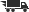Close

# Making Sense of Intermediate Algebra: Models, Functions and Graphs

## by Kysh, Judith

• ISBN: 9780201499957
• ISBN10: 0201499959

# Making Sense of Intermediate Algebra: Models, Functions and Graphs

## by Kysh, Judith

• List Price: \$80.00
• Binding: Paperback
• Edition: 1
• Publisher: Addison Wesley Publishing Company
• Publish date: 01/01/2002
• ISBN: 9780201499957
• ISBN10: 0201499959
used \$13.22
You save: 83%
new \$120.10
Description: 1. Problem Solving. Problem-solving strategies: Using "guess and check" tables, writing equations, graphing data, solving subproblems. Solving linear and quadratic equations. Introduction to the function concept. 2. Functions. Function notation, domain and range. Using a graphing calculator to investigate functions. Fitting lines to data. Writing equations for lines using slope and intercepts. Finding x- and y-intercepts and intersection points for lines by solving linear equations. Finding x- and y- intercepts for parabolas by solving quadratic equations. 3. Sequences as Discrete Functions. Developing sequences from data. Graphing sequences. Using formulas for th terms in arithmetic and geometric sequences. Definitions of continuous versus discrete functions. Relating arithmetic sequences to linear functions. Review of solving systems of equations. 4. Exponential Functions. Seeing geometric sequences as discrete exponential functions. Using integer and noninteger exponents. Relating fractional exponents and radicals. Introduction to solving exponential equations. Writing exponential equations to represent growth or decay. 5. Translating Graphs of Parabolas and Other Functions. Definition of a function. Using the quadratic equation to identify the location of the vertex and the stretch factor for the graph of a parabola. Changing quadratic expressions from standard form to graphing form. Generalizing relationships between equations and graphs for cubic, exponential, and square-root functions. Writing equations for parabolas given their graphs. Introduction to non-functions: parabolas of the form x = y2. 6. Linear Systems. Solving systems of inequalities. Applications of linear inequalities. Solving systems of three equations in three variables. Writing equations for parabolas given three points. 7. Logarithms and Other Inverse Functions. Definition of inverse function. Finding the inverse function (or relation) for a given function. Relating inverse functions and their graphs. Definition of logarithms as inverse functions of exponential functions. Translating the graphs of logarithms. The laws of logarithms. Using logarithms to solve exponential equations. 8. Polynomials and General Systems of Equations. Solving systems graphically. Determining when systems can be solved algebraically. Solving systems of linear and quadratic equations. Complex numbers and their relation to graphs that do not intersect in the xy-plane. Operations with complex numbers. Graphs of polynomial functions. Roots of polynomial functions. Writing equations for polynomial functions given their roots and one other point. Appendix A. Solving Equations. Appendix B. Completing the Square. Appendix C. Circles. Appendix D. 3 x 3 Technology. Index
Expand description
 Seller: Media Smart ★★★★★ ★★★★★ Location: Hawthorne, CA Condition: NewP 400. P 400. Price: \$120.10 Comments: P 400. P 400.